Search
•Aditya Anand

# Breaking Down : SHA-256 Algorithm

#### Looking under the hood and understanding how it works?Good news folks the article that I wrote on Breaking down: SHA-1 Algorithm has been published on PenTest Magazine’s blog. It is always nice to see your work being recognized and appreciated. I am keeping my articles free for everyone to read as I believe in the “knowledge should be free” motto. Well, let’s not dwell into that and get started with the new article.

So, few of you who might be following me for some time now must be knowing that this month I have dedicated to writing articles that are purely focused on doing intricate analysis of how the most well-known hashing algorithms function and what makes one more complex than the other. Till now the articles I have written in this series are as follows.

Breaking Down : The series

1. Breaking Down : MD5 Algorithm

2. Breaking Down: SHA-1 Algorithm

3. Breaking Down : SHA-512 Algorithm

This is the fourth part of the series where I break down, SHA-256 algorithm. Understanding the SHA-256 algorithm will be extremely easy if you know the SHA-512 algorithm already, as there is mere changes in the length of bits here and there as the overall process is the same. If you want you can have a look at my article explaining SHA-512 in detail here.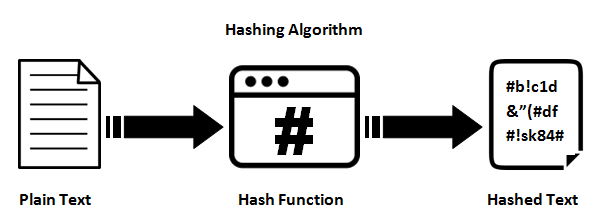### Let’s begin!

So, let us first start this by segregating and defining what are the parts of the computation that we need to carry out one after the another. I personally prefer to break it down into five parts, for the ease of understanding.

### 1. Append : Padding bits

First step of our hashing function begins with appending bits to our original message so that its length will be same to the standard length required for the hash function. To do so we proceed by adding few bits to the message that we have in hand. The number of bits we add is calculated as such so that after addition of these bits the length of the message should be exactly 64 bits less than a multiple of 512. Let me depict it to you in mathematical terms for better understanding.

`M + P + 64 = n x 512`
```i.e M = length of original message

The bits that we append to the message, should begin with ‘1’ and the following bits must be ‘0’ till we are exactly 64 bits less than the multiple of 512.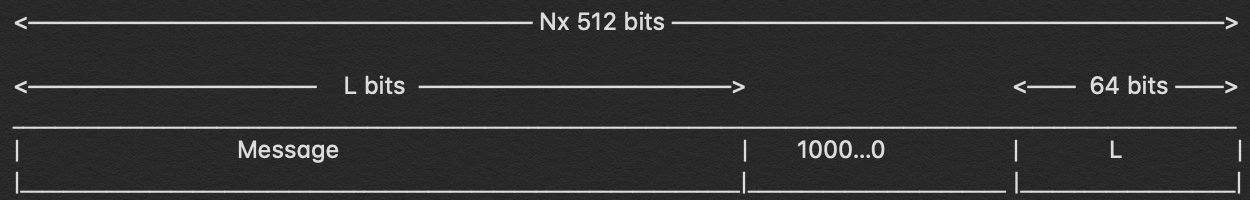### 2. Append : Length bits

Now that we have appended our padding bits to the original message we can further go ahead append our length bits which is equivalent to 64 bits, to the overall message to make the entire thing an exact multiple of 512.

We know that we need to add 64 more bits, the way to calculate these 64 bits is by calculating the modulo of the original message i.e. the one without the padding, with 2³². The message we obtain we append those lengths to the padded bits and we get the entire message block, which must be a multiple of 512.

### 3. Initialize the buffers

We have our message block on which we will begin to carry out our computations to figure out the final hash. Before we begin with that I should tell you that we need certain default values to be initialized for the steps that we are going to perform.

```a = 0x6a09e667
b = 0xbb67ae85
c = 0x3c6ef372
d = 0xa54ff53a
e = 0x510e527f
f = 0x9b05688c
g = 0x1f83d9ab
h = 0x5be0cd19```

Keep these values in the back of your mind for a while, in the next step everything will be clearly understandable to you. There are more 64 values that need to be kept in mind which will act as keys and are denoted by the word ‘k’.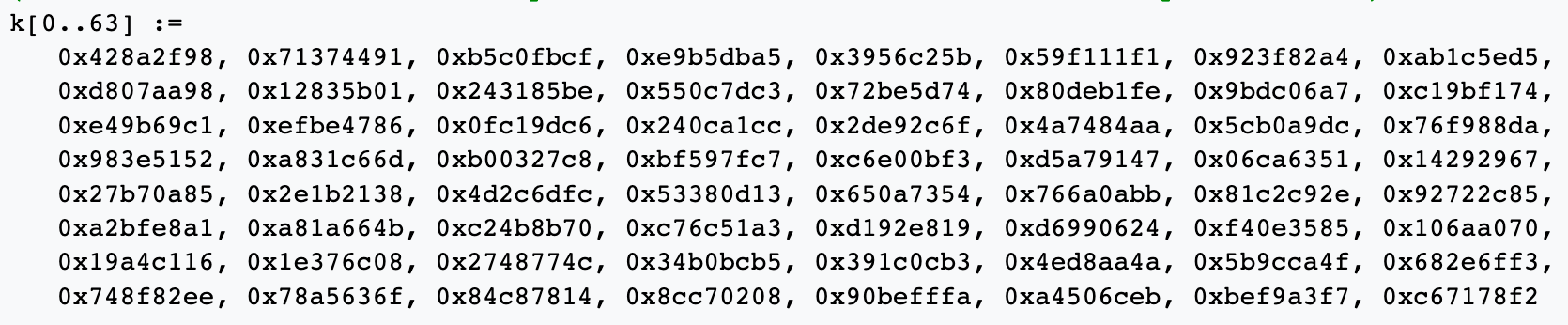Now let’s get into the part where we utilize these values to compute the hash.

### 4. Compression Function

So, the main part of the hashing algorithm lies in this step. The entire message block that we have ‘n x 512’ bits long is divided into ‘n’ chunks of 512 bits and each of these 512 bits, are then put through 64 rounds of operations and the output obtained is fed as input for the next round of operation.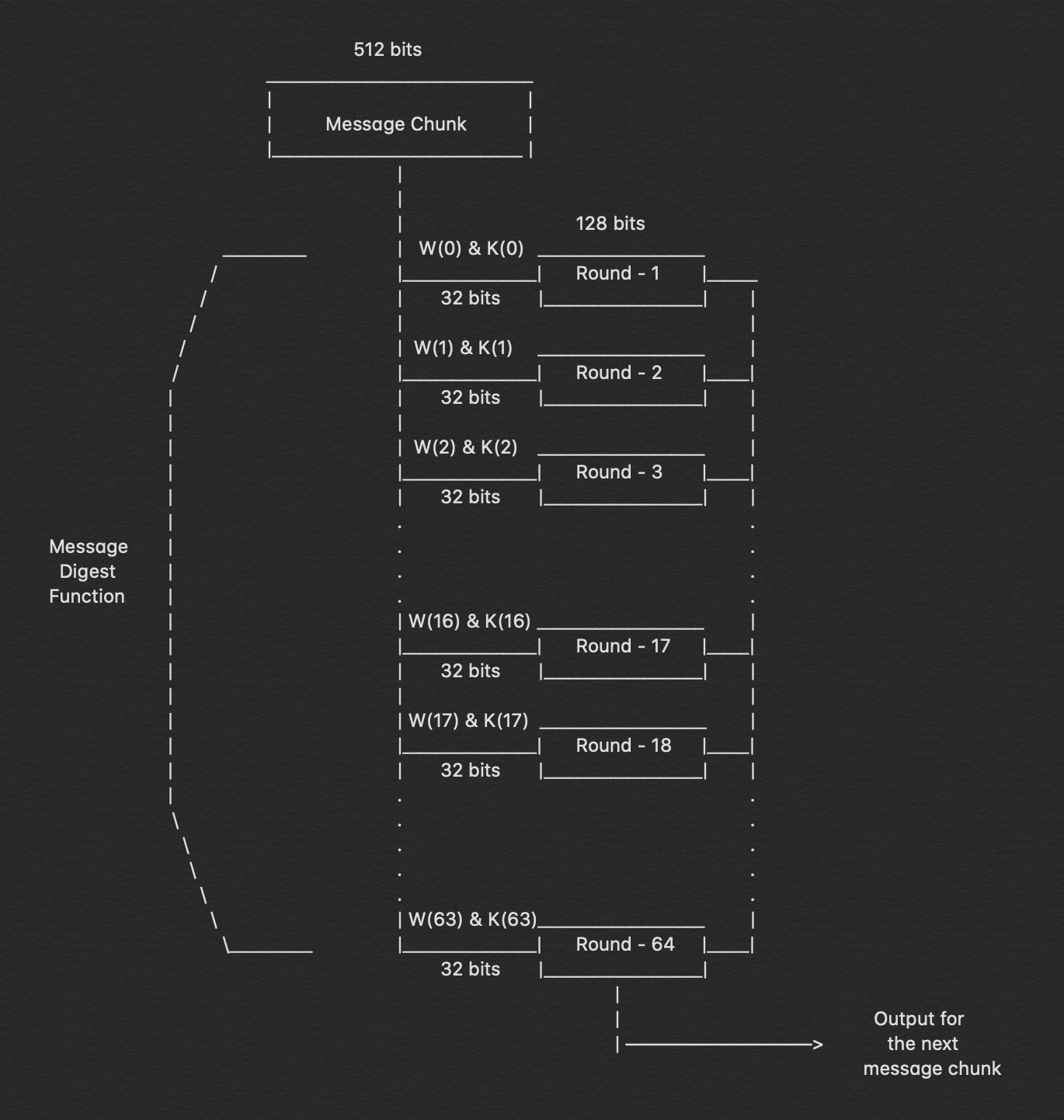In the image above we can clearly see the 64 rounds of operation that is performed on a 512-bit message. We can observe that two inputs that we send in are W(i) & K(i), for the first 16 rounds we further break down the 512-bit message into 16 parts each of 32 bit but after that, we need to calculate the value for W(i) at each step.

`W(i) = Wⁱ⁻¹⁶ + σ⁰ + Wⁱ⁻⁷ + σ¹`
```where,
σ⁰ = (Wⁱ⁻¹⁵ ROTR⁷(x)) XOR (Wⁱ⁻¹⁵ ROTR¹⁸(x)) XOR (Wⁱ⁻¹⁵ SHR³(x))
σ¹ = (Wⁱ⁻² ROTR¹⁷(x)) XOR (Wⁱ⁻² ROTR¹⁹(x)) XOR (Wⁱ⁻² SHR¹⁰(x))
ROTRⁿ(x) = Circular right rotation of 'x' by 'n' bits
SHRⁿ(x)  = Circular right shift of 'x' by 'n' bits```

Well now that we have a well-established method to create the W(i) for any given of the 64 rounds let’s dive in what happens in each of these rounds.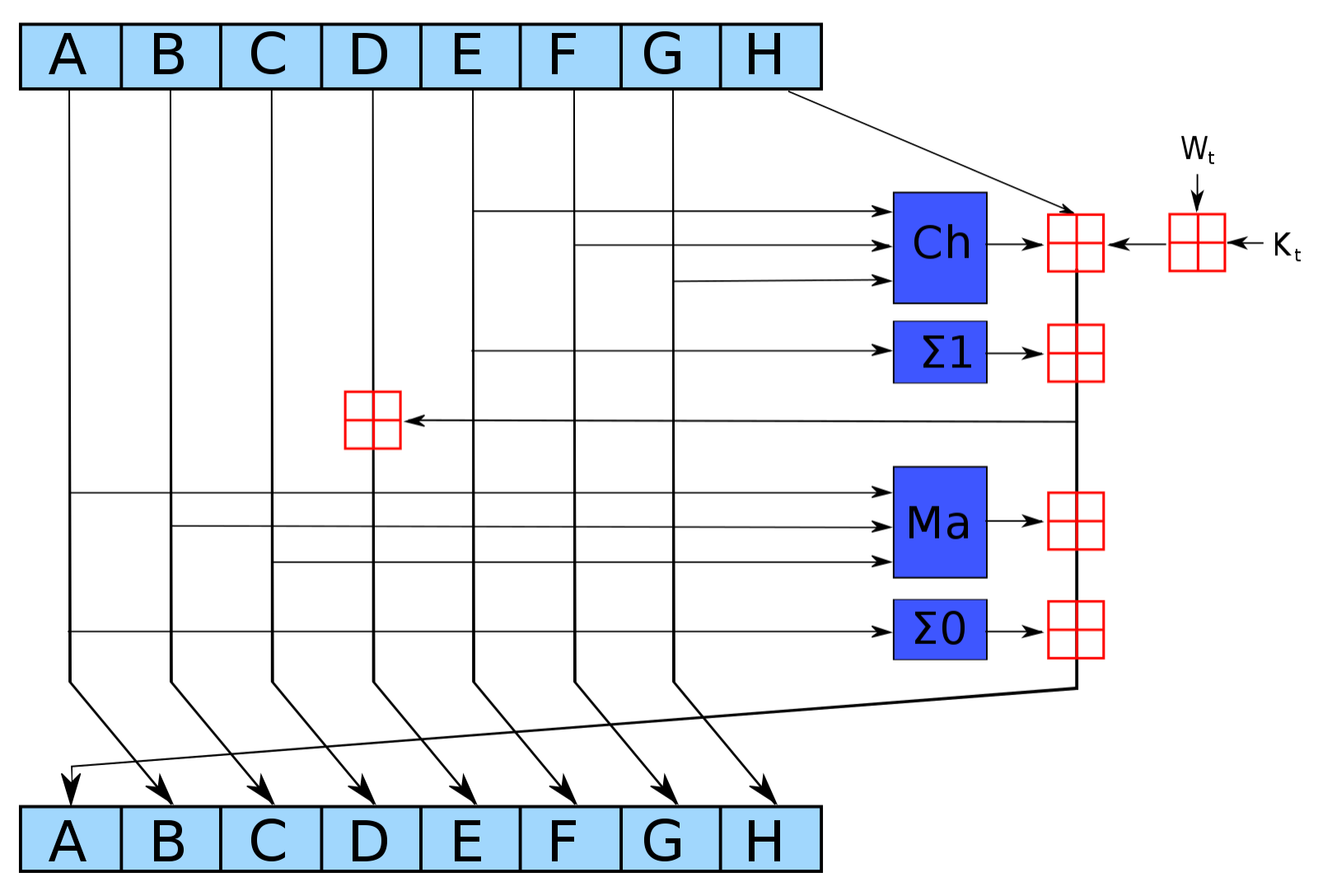In the image above we can see exactly what happens in each round and now that we have the values and formulas for each of the functions carried out we can perform the entire hashing process.

```Ch(E, F, G) = (E AND F) XOR ((NOT E) AND G)
Ma(A, B, C) = (A AND B) XOR (A AND C) XOR (B AND C)
∑(A)    = (A >>> 2) XOR (A >>> 13) XOR (A >>> 22)
∑(E)    = (E >>> 6) XOR (E >>> 11) XOR (E >>> 25)

These are the functions that are performed in each of the 64 rounds that are performed over and over for ‘n’ number of times

### 5. Output

The output from every round act as an input for the next round and this process keeps on continuing till the last bits of the message remains and the result of the last round for the nᵗʰ part of the message block will give us the result i.e. the hash for the entire message. The length of the output is 256 bits.

### Conclusion

The SHA-256 hashing algorithm is currently one of the most widely used hashing algorithms as it hasn’t been cracked yet and the hashes are calculated quickly in comparison to the other secure hashes like the SHA-512. It is very well established but the industry is trying to slowly move towards the SHA-512 which is more secure as experts claim SHA-256 might be vulnerable very soon.

So, let’s have a second look at the entire functioning of the SHA-256 algorithm and allow me to explain the entire thing in a single long paragraph.

We calculate the length of the message that needs to be hashed, we then append few bits to the message, starting with ‘1’ and the rest are ‘0’ till the point the message length is exactly 64 bits less than the multiple of 512. We add the remaining 64 bits by calculating the modulo of the original message with 2³². Once, we add the remaining bits the entire message block can be represented as ‘n x 512’ bits. Now, we pass each of these 512 bits into the compression function i.e. the set of 64 rounds of operations where we further divide them into 16 parts each of 32 bits. These 16 parts each of 32 bits act as input for each round of operation for the first 16 rounds and for the rest of the 48 rounds we have a method to calculate the W(i). We also have default values for the buffers and the values of ‘k’ for all the 64 rounds. We can now begin the computation of hashes as we have all the values and formulas required. The hashing process is then carried out on over and over for 64 rounds and then the output of i round works as input for the i+1 round. So the output from the 64ᵗʰ operation of the nᵗʰ round will present us with the output i.e. the hash of the entire message.

So that’s the short version of the entire operation that takes place in the SHA-256 algorithm.

If you enjoyed it please do clap & let’s collaborate. Get, Set, Hack!